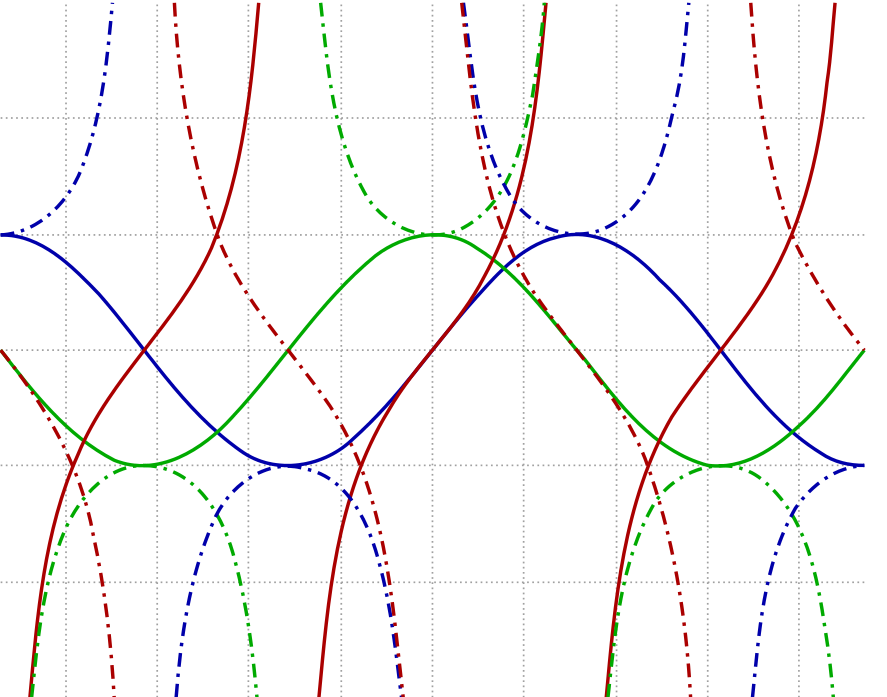# Graphometry

Geometry Level 1$\large \begin{cases}{y=\sin (x)} \\ {y=\cos (x)} \\ {y=\tan (x)} \\ {y=\csc (x)}\end{cases}$

The four graphs above are drawn on the same axes from $x = 0$ to $x = \frac \pi2$.

If a vertical line is drawn where the graphs of $y=\cos (x)$ and $y = \tan (x)$ intersect, this line intersects the graphs of $y=\sin(x)$ and $y=\csc(x)$ at points $A$ and $B$. What is the distance between $A$ and $B$?

×